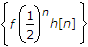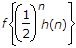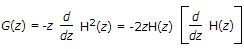# Electronics and Communication Engineering - Exam Questions Papers - Discussion

Discussion Forum : Exam Questions Papers - Exam Paper 4 (Q.No. 31)
31.
Consider a stable and causal system with impulse response h[n] and rational system function H(z). Suppose it is known that H(z) contains a pole at z = 1/2 and a zero some where on the unit circle. The precise number and locations of all of the other poles is unknown.
The following statement which is false is __________ .
H(e) = 0 for some ωg[n] = {n[h[n * h[n]} is the impulse response of a stable system
h[n] has a finite duration
Explanation:

Statement (d) is false because a finite-duration sequence must have an ROC that includes the entire z-plane, except possible z = 0 and/or z = ∞

This is not consistent with having a pole at z = 1/2.

Statement (b) is truecorresponds to the value of the z-transform of h[n] at z = 2.

Thus, its convergence is equivalent to the point z = 2 being in the ROC.

Since the system is stable and causal, all of the poles of H(z) are inside the unit circle, and the ROC includes all the points outside the unit circle, including z = 2.

Statement (c) is true. Since the system is causal, h[n] = 0 for n < 0.

Consequently, h[n] * h[n] = 0 for n < 0; i.e., system with h[n] * h[n] as its impulse response is causal.

The same is then true for g[n] = n[h[n]].

Furthermore, by the convolution property the system function corresponding to the impulse response h[n] * h[n] is H2(z), and by the differentiation property the system function corresponding to g[n] isFrom above equation we can conclude that the poles of G(z) are at the same locations as those of H(z), with the possible exception of the origin.

Therefore, since H(z) has all its poles inside the unit circle, so must G(z).

It follows that g[n] is the impulse response of a causal and stable system.

Statement (a) is true because there is a zero on the unit circle.

Discussion:
Be the first person to comment on this question !